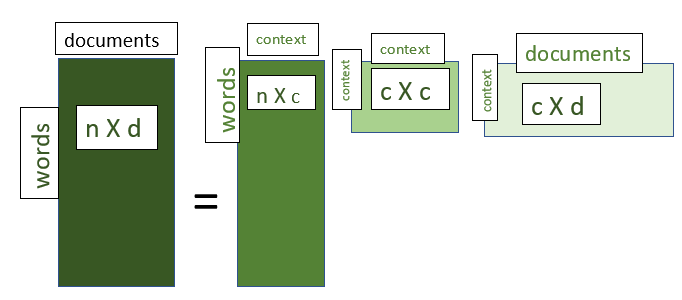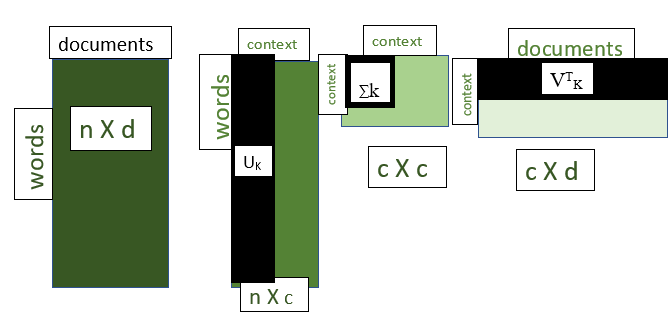# Latent Semantic Analysis

• Difficulty Level : Medium
• Last Updated : 30 May, 2021

Latent Semantic Analysis is a natural language processing method that uses the statistical approach to identify the association among the words in a document. LSA deals with the following kind of issue:

Example: mobile, phone, cell phone, telephone are all similar but if we pose a query like “The cell phone has been ringing” then the documents which have “cell phone” are only retrieved whereas the documents containing the mobile, phone, telephone are not retrieved.

Assumptions of LSA:

1. The words which are used in the same context are analogous to each other.
2. The hidden semantic structure of the data is unclear due to the ambiguity of the words chosen.

Singular Value Decomposition:

Singular Value Decomposition is the statistical method that is used to find the latent(hidden) semantic structure of words spread across the document.

Let

```C = collection of documents.
d = number of documents.
n = number of unique words in the whole collection.
M = d X n ```

The SVD decomposes the M matrix i.e word to document matrix into three matrices as follows

M = U∑VT

where

```U = distribution of words across the different contexts
∑ = diagonal matrix of the association among the contexts
VT = distribution of contexts across the different documents```SVD OF n x d matrix

A very significant feature of SVD is that it allows us to truncate few contexts which are not necessarily required by us. The ∑ matrix provides us with the diagonal values which represent the significance of the context from highest to the lowest. By using these values we can reduce the dimensions and hence this can be used as a dimensionality reduction technique too.

If we select the k the largest diagonal values in ∑ a matrix we obtain

Mk = UkkVTK

where

```Mk = approximated matrix of M
Uk, ∑k, VTk are the matrices containing  only the k contexts from U, ∑, VT respectively```Truncated SVD after selecting k value

My Personal Notes arrow_drop_up